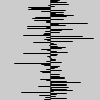Name

randomGaussian()

Examplesfor y in range(100):
x = randomGaussian() * 15
line(50, y, 50 + x, y)
distribution = [0.0] * 360
size(100, 100)
for i in range(len(distribution)):
distribution[i] = int(randomGaussian() * 15)
background(204)
translate(width / 2, width / 2)
for i in range(len(distribution)):
rotate(TWO_PI / len(distribution))
stroke(0)
dist = abs(distribution[i])
line(0, 0, dist, 0)
Description Returns a float from a random series of numbers having a mean of 0 and standard deviation of 1. Each time the randomGaussian() function is called, it returns a number fitting a Gaussian, or normal, distribution. There is theoretically no minimum or maximum value that randomGaussian() might return. Rather, there is just a very low probability that values far from the mean will be returned; and a higher probability that numbers near the mean will be returned.
Syntax
randomGaussian()
Related random()
noise()

Updated on Mon Sep 21 15:53:25 2020.

If you see any errors or have comments, please let us know.